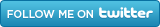## Search This Blog## Saturday, 16 September 2017

### ProbablePrejudice

Think about this game:  suppose you have an urn filled with equal numbers of red and green marbles.  You reach in and take out a marble in your clenched hand so you can't see it.  What colour do you guess the marble is if you want to be right as often as possible?  The answer is it doesn't matter.  If you guess red or green at the toss of a coin you will score 50%.  If you always guess green you will also score 50%.  The same goes for any proportion of guesses in between.

But this cannot be true if there are more red marbles than green.  In the extreme, if there are only red marbles in the urn, you would clearly be crazy ever to guess green.  So what is the general rule if the proportion of red marbles is p and you know the value of p?

Suppose the proportion of red guesses you make is r.  Should r = p?  That seems to be true from the argument above if p = 1, and maybe if p = 0.5.  But it may not be true if p = 0.8, say.  Let's look at the sums:

A red marble comes out of the urn a fraction p of the time.  If you guess red r of the time you will be right pr of the total time for those red marbles.

A green marble comes out of the urn (1 - p) of the time.  If you guess green (1 - r) of the time you will be right (1 - p)(1 - r) of the total time for those green marbles.

So the total proportion of correct guesses you make, c, is

c = pr + (1 - p)(1 - r)

= r(2p - 1) - p +1

If we plot a graph of correct guesses, c, for different values of r when p = 0.5 we get:

Which tells us what we said when we started - for equal numbers of reds and greens it doesn't matter what proportion of red guesses, r, you make, you will always score c = 50%.

But now suppose p = 0.8 (that is, 80% of the marbles in the urn are red).  Then the graph does this:

Now what is the best guessing strategy, r, to give the biggest value of correct guesses, c? It is not r = p as we conjectured.  It is to guess red all the time.  This gives a highest possible score of 80%.

This happens even for the tiniest majority of red or green marbles.  If you know red is in the majority, no matter how small that majority is, you always guess red.  If you know green is in the majority you always guess green.

This is a really easy rule for evolution to encode: if there are two types of things, A and B, and you know that A are in the majority, then - when encountering a thing with no other knowledge - assume the thing is A.  You will be right as often as it is possible to be.

A and B might be bears and tigers growling out of sight.  If you know there are more bears than tigers, then your best bet is to assume you have to deal with a bear.

This argument affects the best way for you to allocate resources.  Suppose that it costs you the same to prepare to encounter an A in the future as it costs to prepare to encounter a B.  Further suppose that the reward (or loss) you get if you meet an A is the same as the reward (or loss) you get if you meet a B.  Then, if there are even just a few more As than Bs, it is optimal to spend ALL your resources on preparing to meet As and to spend NONE on preparing to meet Bs.

Of course, A and B might not be bears and tigers; they might be people of unknown sexuality, nationality, or (if - like the bears and tigers - they are also out of sight) gender or ethnicity...